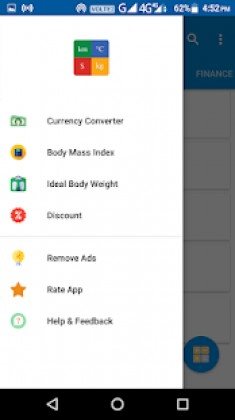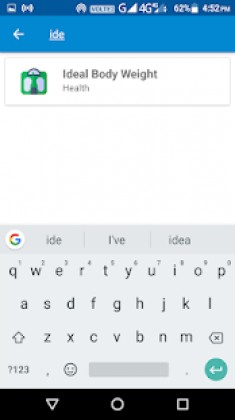All-In-One Calculator and Unit Converter (PRO) 3 Apk latest | Download Android

All-In-One Calculator and Unit Converter (PRO) 3 Apk latest

event_note01 Aug 2018
android Apps, Tools

All-In-One Calculator and Unit Converter (PRO) 3 Apk latest is a Tools Android app

Download last version All-In-One Calculator and Unit Converter (PRO) Apk For Android with direct link

All-In-One Calculator and Unit Converter (PRO) is a Tools android app made by Samved that you can install on your android devices an enjoy !

All-in-one Calculator and Unit Converter is an easy to use app with features that you need.

This app included 15+ Unit Converters, Ideal Body Weight Calculator, Tip Calculator and many other features that you will love.

List of features that are supported –

1) General Calculator – Supports general mathematical operations such as addition, subtraction, multiplication, division, square root, and power

2) Algebra Calculator – Supports Equation Solver, Fractions, GCF / LCM, Percentage

3) Unit Converter – Supports conversion of units of Angle, Area, Data Storage, Data Transfer, Energy, Length, Number System, Pressure, Shoe Size, Speed, Temperature, Time, Volume, Weight

4) Health Calculators – Supports Basal Metabolic Rate (BMR), Body Mass Index (BMI), Body Fat Percentage, Calorie Intake, Ideal Body Weight

5) Finance Calculators – Supports Compound Interest, Discount, Simple Interest, Tip Calculator

6) Geometry Calculators – Supports Perimeter, Area, Volume, Surface Area Calculators along with Heron’s Formula Calculator

7) Time Calculators – Age Calculator, Elapsed Time Calculator (for both dates and times)All-In-One Calculator and Unit Converter (PRO) ApkAll-In-One Calculator and Unit Converter (PRO) Apk

Whats New:

* Bug fixes